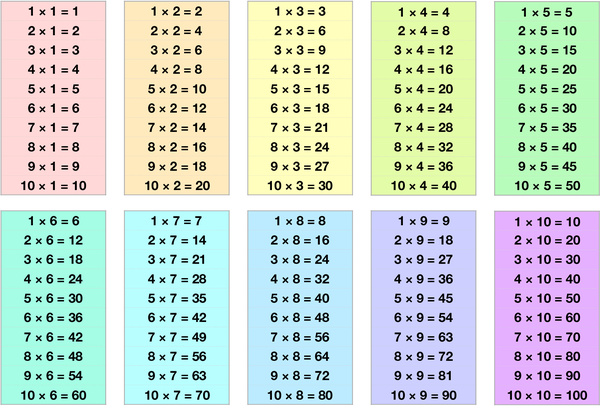# Multiplication Table 10x10Memorizing the multiplication tables up to 10x10 is an important foundational skill in mathematics. Learning the times table up to 10 by 10 is an essential skill for children as it provides a foundation for more complex mathematical concepts they will encounter later in their education. Here are some reasons why it's important:

Builds strong foundational skills: Multiplication is a fundamental concept in mathematics, and learning the times table up to 10 by 10 provides a solid foundation for future mathematical concepts.

Helps with mental math: Knowing the times tables can help children do mental calculations more quickly and accurately, which can be a valuable skill in daily life.

Improves problem-solving skills: Multiplication is an essential skill for problem-solving, and children who know the times table up to 10 by 10 will be better equipped to solve mathematical problems.

Increases confidence: Children who have a strong understanding of the times table are more likely to feel confident in their mathematical abilities, which can positively impact their academic performance.

Prepares for advanced math: Knowing the times table up to 10 by 10 is a prerequisite for learning more complex mathematical concepts, such as fractions, decimals, and algebra.

Overall, learning the times table up to 10 by 10 is an important foundational skill that will benefit children both in their academic and daily lives. Here are the times tables up to 10x10:

1 x 1 = 1
1 x 2 = 2
1 x 3 = 3
1 x 4 = 4
1 x 5 = 5
1 x 6 = 6
1 x 7 = 7
1 x 8 = 8
1 x 9 = 9
1 x 10 = 10

2 x 1 = 2
2 x 2 = 4
2 x 3 = 6
2 x 4 = 8
2 x 5 = 10
2 x 6 = 12
2 x 7 = 14
2 x 8 = 16
2 x 9 = 18
2 x 10 = 20

3 x 1 = 3
3 x 2 = 6
3 x 3 = 9
3 x 4 = 12
3 x 5 = 15
3 x 6 = 18
3 x 7 = 21
3 x 8 = 24
3 x 9 = 27
3 x 10 = 30

4 x 1 = 4
4 x 2 = 8
4 x 3 = 12
4 x 4 = 16
4 x 5 = 20
4 x 6 = 24
4 x 7 = 28
4 x 8 = 32
4 x 9 = 36
4 x 10 = 40

5 x 1 = 5
5 x 2 = 10
5 x 3 = 15
5 x 4 = 20
5 x 5 = 25
5 x 6 = 30
5 x 7 = 35
5 x 8 = 40
5 x 9 = 45
5 x 10 = 50

6 x 1 = 6
6 x 2 = 12
6 x 3 = 18
6 x 4 = 24
6 x 5 = 30
6 x 6 = 36
6 x 7 = 42
6 x 8 = 48
6 x 9 = 54
6 x 10 = 60

7 x 1 = 7
7 x 2 = 14
7 x 3 = 21
7 x 4 = 28
7 x 5 = 35
7 x 6 = 42
7 x 7 = 49
7 x 8 = 56
7 x 9 = 63
7 x 10 = 70

8 x 1 = 8
8 x 2 = 16
8 x 3 = 24
8 x 4 = 32
8 x 5 = 40
8 x 6 = 48
8 x 7 = 56
8 x 8 = 64
8 x 9 = 72
8 x 10 = 80

9 x 1 = 9
9 x 2 = 18
9 x 3 = 27
9 x 4 = 36
9 x 5 = 45
9 x 6 = 54
9 x 7 = 63
9 x 8 = 72
9 x 9 = 81
9 x 10 = 90

10 x 1 = 10
10 x 2 = 20
10 x 3 = 30
10 x 4 = 40
10 x 5 = 50
10 x 6 = 60
10 x 7 = 70
10 x 8 = 80
10 x 9 = 90
10 x 10 = 100